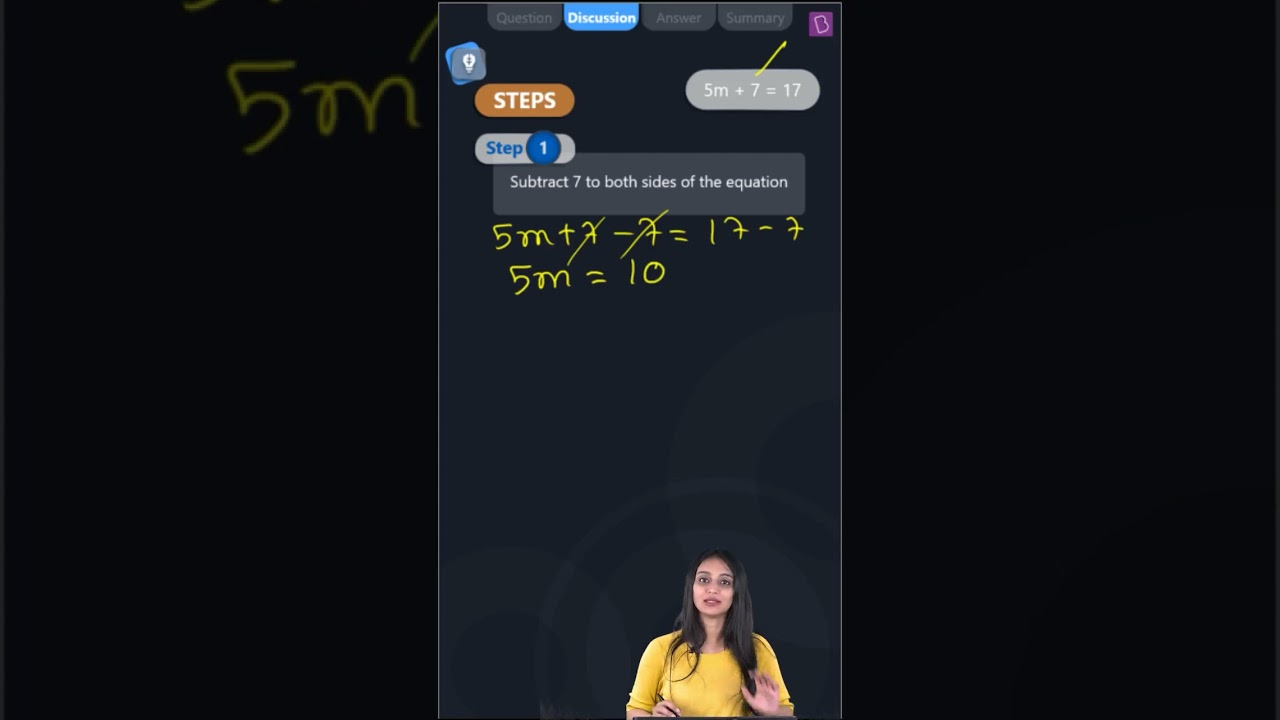Give the steps you will use to separate the variable and then solve the equation: 5m + 7 = 17Given equation: 5m + 7 = 17

First, we have to subtract 7 to both sides of the equation,

Then, we get,

⇒ 5m + 7 – 7 = 17 – 7

⇒ 5m = 10

Now,

We have to divide both sides of the equation by 5,

Then, we get,

⇒ 5m/5 = 10/5

m = 2(3)(1)